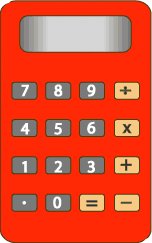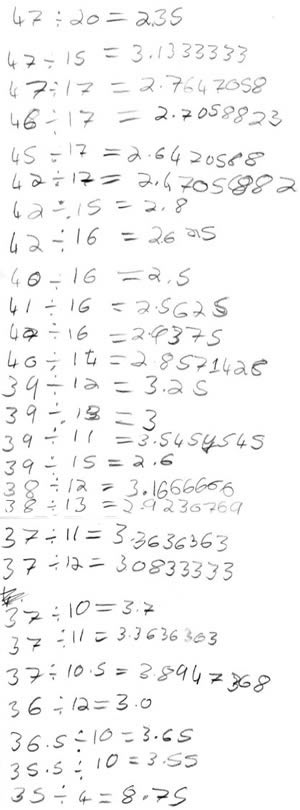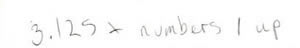#### You may also like### Pebbles

Place four pebbles on the sand in the form of a square. Keep adding as few pebbles as necessary to double the area. How many extra pebbles are added each time?### Bracelets

Investigate the different shaped bracelets you could make from 18 different spherical beads. How do they compare if you use 24 beads?### Sweets in a Box

How many different shaped boxes can you design for 36 sweets in one layer? Can you arrange the sweets so that no sweets of the same colour are next to each other in any direction?

# Forgot the Numbers

##### Age 7 to 11Challenge Level

If you are a teacher click here for a version of the problem suitable for classroom use, together with supporting materials. Otherwise, read on ...On my calculator I divided one whole number by another whole number and got the answer $3.125$.

I know that both numbers were less than $50$, but can't remember what they were.

Can you work out what they were?

Once you've had a chance to think about it, click below to see how four different pupils began working on the task.

Here is Gemma and Flo's work:Richard wrote the following:He explained:

"I multiplied $3.125$ by $1$, then I tried multiplying $3.125$ by $2$, then I multiplied $3.125$ by $3$ ..."

Here is the start of Thomas' work:

I first looked at the number $0.125$ and worked out what fraction of $1$ it is. It turned out that it was an eighth.

Can you take each of these starting ideas and develop it into a solution?